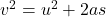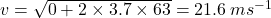## A car accelerates uniformly in a straight line from rest at the rate of 3.7 m/s2 What is the speed of the car after it has traveled 63

Question

A car accelerates uniformly in a straight line from rest at the rate of 3.7 m/s2
What is the speed of the car after it has traveled 63 m? Answer in units of m/s.
How long does it take the car to travel 63 m? Answer in units of s

in progress 0
6 months 2021-07-13T05:12:00+00:00 1 Answers 0 views 0

## Answers ( )

1. Explanation:

Using equations of motion :

(A) we know that,

u = 0

a = 3.7 m/s^2

S = 63(B)

v = u + at

21.6 = 0 + 3.7 * t

t (time to reach 63 m) = 5.83 seconds